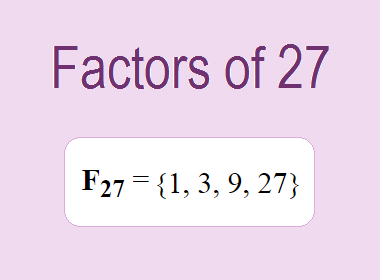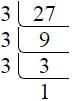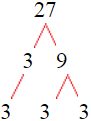# Factors of 27The factors of 27 are 1, 3, 9, and 27 i.e. F27 = {1, 3, 9, 27}. The factors of 27 are those numbers that can divide 27 without leaving a remainder.

We can check if these numbers are factors of 27 by dividing 27 by each of them. If the result is a whole number, then the number is a factor of 27. Let's do this for each of the numbers listed above:

·        1 is a factor of 27 because 27 divided by 1 is 27.

·        3 is a factor of 27 because 27 divided by 3 is 9.

·        9 is a factor of 27 because 27 divided by 9 is 3.

·        27 is a factor of 27 because 27 divided by 27 is 1.

## How to Find Factors of 27?

1 and the number itself are the factors of every number. So, 1 and 27 are two factors of 27. To find the other factors of 27, we can start by dividing 27 by the numbers between 1 and 27. If we divide 27 by 2, we get a remainder of 1. Therefore, 2 is not a factor of 27. If we divide 27 by 3, we get a remainder of 0. Therefore, 3 is a factor of 27.

Next, we can check if 4 is a factor of 27. If we divide 27 by 4, we get a remainder of 3. Therefore, 4 is not a factor of 27. We can continue this process for all the possible factors of 27.

Through this process, we can find that the factors of 27 are 1, 3, 9, and 27. These are the only numbers that can divide 27 without leaving a remainder.

********************

********************

## Properties of the Factors of 27

The factors of 27 have some interesting properties. One of the properties is that the sum of the factors of 27 is equal to 40. We can see this by adding all the factors of 27 together:

1 + 3 + 9 + 27 = 40

Another property of the factors of 27 is that they are all odd numbers. This is because 27 is an odd number, and any even number cannot divide an odd number without leaving a remainder.

Another property of the factors of 27 is that the only prime factor of 27 is 3.

## Applications of the Factors of 27

The factors of 27 have several applications in mathematics. One of the applications is in finding the highest common factor (HCF) of two or more numbers. The HCF is the largest factor that two or more numbers have in common. For example, to find the HCF of 27 and 45, we need to find the factors of both numbers and identify the largest factor they have in common. The factors of 27 are 1, 3, 9, and 27. The factors of 45 are 1, 3, 5, 9, 15, and 45. The largest factor that they have in common is 9. Therefore, the HCF of 27 and 45 is 9.

Another application of the factors of 27 is in prime factorization. Prime factorization is the process of expressing a number as the product of its prime factors. The only prime factor of 27 is 3. We can express 27 as:

27 = 3 × 3 × 3

We can do prime factorization by division and factor tree method also. Here is the prime factorization of 27 by division method,27 = 3 × 3 × 3

Here is the prime factorization of 27 by the factor tree method,27 = 3 × 3 × 3

## Conclusion

The factors of 27 are the numbers that can divide 27 without leaving a remainder. The factors of 27 are 1, 3, 9, and 27. The factors of 27 have some interesting properties, such as being odd numbers and having a sum of 40. The factors of 27 have several applications in mathematics, such as finding the highest common factor and prime factorization.